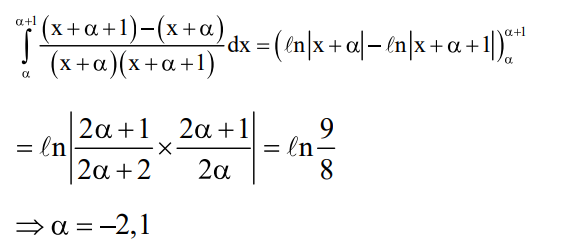# A value of α such that`
Question:

A value of $\alpha$ such that

$\int_{\alpha}^{\alpha+1} \frac{d x}{(x+\alpha)(x+\alpha+1)}=\log _{e}\left(\frac{9}{8}\right)$ is :

1. $\frac{1}{2}$

2.  2

3. $-\frac{1}{2}$

4. $-2$

Correct Option: , 4

Solution: